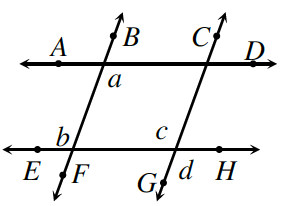### Home > GC > Chapter 7 > Lesson 7.2.6 > Problem7-96

7-96.

Penn started the proof below to show that if $\overline{AD}//\overline{EH}$ and $\overline{BF}//\overline{CG}$, then $a=d$. Unfortunately, he did not provide reasons for his proof. Copy his proof and provide justification for each statement.

For each numbered statement, write how you know it to be true. Think about how angles and parallel lines relate to each other.

Statements

Reasons

1. $\overline{AD}//\overline{EH}$ and $\overline{BF}//\overline{CG}$

Given information.

1. $a=b$

When lines are parallel, then the alternate interior angles are congruent.

1. $b=c$

1. $a=c$

1. $c=d$

1. $a=d$